# Class 9 RD Sharma Solutions – Chapter 23 Graphical Representation of Statistical Data – Exercise 23.2 | Set 2

• Last Updated : 18 Mar, 2021

### Question 11. The following data gives the amount of manure (in a thousand tonnes) manufactured by a company during some years:

(i) Represent the above data with the help of a bar graph.

(ii) Indicate with the help of the bar graph the year in which the amount of manure manufactured by the company was maximum.

(in) Choose the correct alternative:

The consecutive years during which there was maximum decrease in manure production are:

(a) 1994 and 1995

(b) 1992 and 1993

(c) 1996 and 1997

(d) 1995 and 1996

Solution:

(i) The following bar graph represents information in the form of horizontal axes and vertical axes representing years and manure (in thousand tonnes) respectively.

(ii) The maximum amount of manure manufactured was 45 thousand tonnes produced in the year 1994.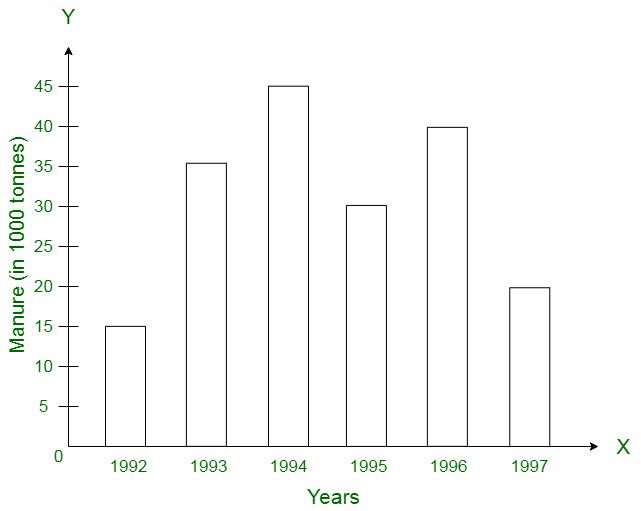(iii) The maximum decrease in manure production was witnessed from the year 1996 to 1997.

### Represent the data with the help of a bar graph. Indicate with the help of the bar graph the course where estimated requirement is least.

Solution:

The following bar graph represents information in the form of horizontal axes and vertical axes representing qualifications and personnel required respectively. The course where requirement expected least is DCE.

### Represent the above data by a bar graph.

Solution: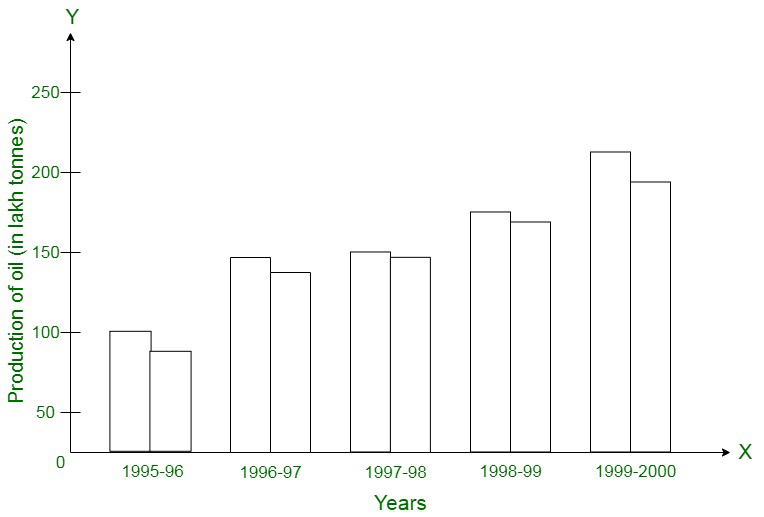### Represent the above data with the help of a bar graph.

Solution: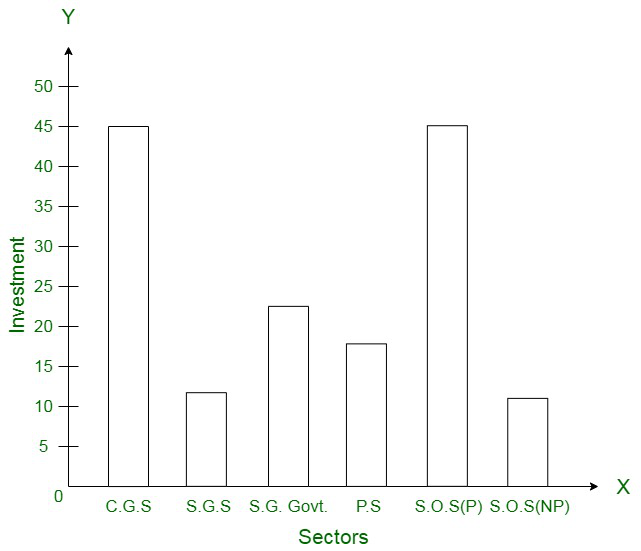### Represent the above data with the help of a bar graph. Indicate with the help of a bar graph the year in which the rate of increase in exports is maximum over the preceding year.

Solution: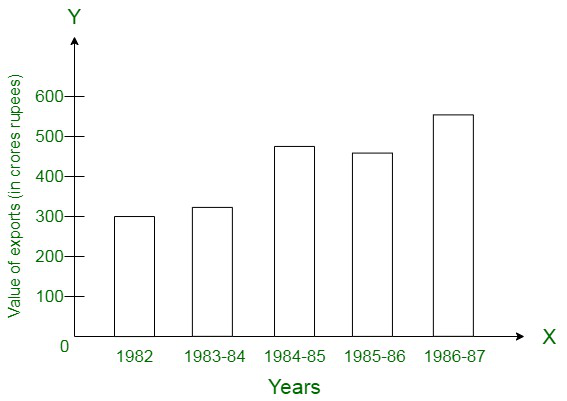### Represent this information with the help of a bar graph.Explain through the bar graph if the quantity of goods carried by the Indian Railways in 1965-66 is more than double the quantity of goods carried in the year 1950-51.

Solution: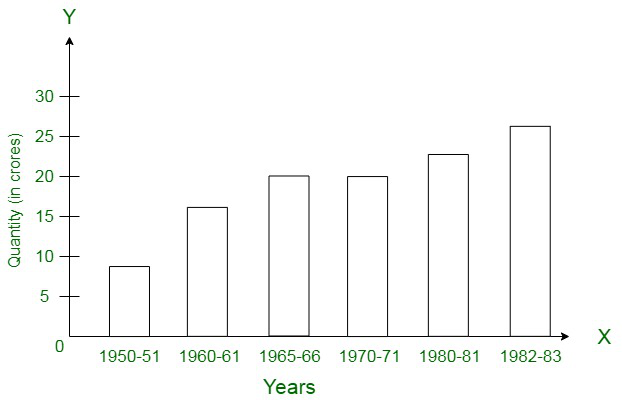### Construct a bar graph to represent the above data so that the bars are drawn horizontally.

Solution: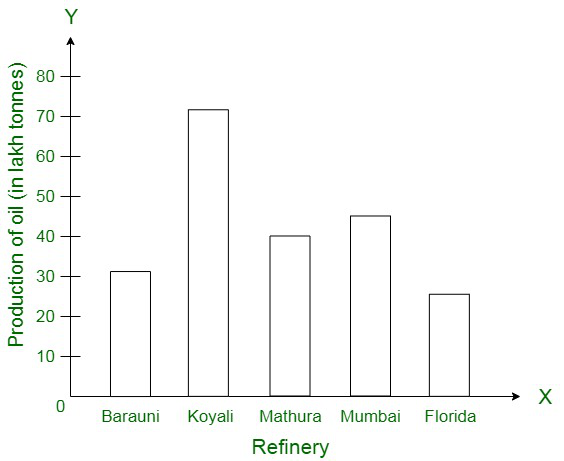### Construct a bar graph to represent the above data.

Solution: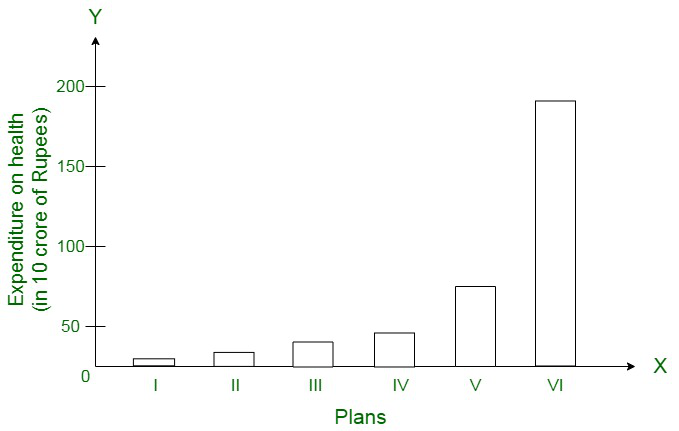My Personal Notes arrow_drop_up